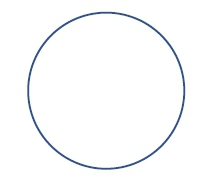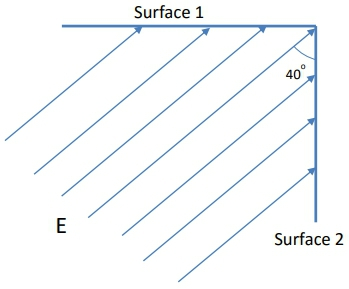## Physics

Learn the toughest concepts covered in Physics with step-by-step video tutorials and practice problems by world-class tutors

24. Electric Force & Field; Gauss' Law

# Electric Flux

1
concept

## Electric Flux5m
Play a video:
2
Problem

The electric flux through each surface of a cube is given below. Which surfaces of the cube does the electric field run parallel to?

Φ1 = 100 Nm2 /C           Φ4 = 0 Nm2 /C
Φ2 = 20 Nm2 /C             Φ5 = −40 Nm2 /?
Φ3 = 0 Nm2 /C               Φ6 = −80 Nm2 /?

3
example

## Flux Through Angled Surface2m
Play a video:
Alright, guys, let's get some more practice with this electric flux stuff. So we have this electric field and we have the area of the surface and we're told to find with the magnitude of the electric flux is through the surface depicted. So let's go ahead and start with the equation. Three. Electric flux is just going to be e magnitude of the electric field times the area times the cosine of the angle feta. Now, if you take a look at this, we actually told what the electric field is. We have that and we're told the area of the surface is just 1 m squared and it's in the right units. So the only thing we have to find is we have to find it with the cosine of the angle is, And what I want you guys to do is remember that we were never We're dealing with co signs of angles, especially with this electric flux. This angle represents the angle between the area or the normal of the surface and the electric field. So if we sort of extend these electric field lines out like this than what we really need to Dio is we need to find what the normal of the surface is. The normal of the surface is always perpendicular to the surface itself. So it points out in that direction. And the reason we're told to find the magnitude of the electric flux is because we actually have no idea. We have no information of whether the normal is pointing this way or this way. So we're just gonna go ahead and assume that it's positive by calculating the magnitude of the electric flux anyways. So the angle that we really need is actually the angle between this vector and the normal of the surface, which is not the same as this 30 degrees. This 30 degrees is the angle between the electric field and the surface itself, not the perpendicular of that surface. So really, we're not using this 30 degrees. Instead, we're just going to use 30 plus 60 equals 90 so this angle is actually 60 degrees, which is this angle as well by the geometry, right? These things are all opposite angles. So what we really need to dio is that the electric flux is going to be 100 Newtons per Coolum. The area is just 1 m squared. And now we have to do just to co sign of 60 degrees. Now, cost of 60 is just one half. So that means the electric flux is just half of 100 which is 50. And that electric flux is Newton meters squared per cool. Um, just in case you needed the units. Alright, so that's the answer. Let me know if you guys have any questions.
4
example

## Flux Through Cube3m
Play a video:
Alright, guys, let's work this one out together. So we have a cube and its side length is two centimeters and it's placed in a uniformed electric fields. We've got two centimeters right here. We're supposed to figure out what the electric flux is through each side of the Cube. So let's go ahead and see what sort of visualize what's going on here. First we're gonna do is gonna draw the normal vectors for each one of those sides. So the top one has a normal vector like this. This one has the one that goes sort of like outwards like this. Sort of like out towards you. Think one has one to the right thing. One's got one to the left and then this one. There's some bottom one that's gonna be all the way down here That's gonna point that way. And there's gonna be a back one sort of on the back side of the Cube. Sort of like we can't see it right now. Um, Anyways, each one of these surfaces now may or may not have an electric field that goes through it, so we know we're gonna have to calculate the electric flux for all of these. So just remember that it's e a co sign of data for the electric flux. Okay, so clearly we can see that for the right side. There is definitely going to be some electric flux here, So the right side is just going to be e times a times the co sine of the angle. But in this case, we have the e A. The cosine of the angle is just going to be zero. And so this is just gonna be e times A. Whereas on the other side, we could basically make the opposite arguments that you have the same exact area because it's a cube. Except in this case, the flux on the left side is just gonna b e a times the co sign of 1 80. And so it's gonna be equal to negative E times a okay, and then everywhere else, let's go ahead and see what's going on for the top side. You have an electric field that points in this direction, and you have a normal vector that points in the upward direction. That means that the co sign of this angle right here that it makes is equal to zero. So that means that over here the flux at the top is just equal to zero. And by the way, if that's the case, then that means the flux in the bottom is also equal to zero because of the same reasoning. And now the only two of the rain on the front and the back side. So now you have an electric field that points in this direction, and now you have an angle, a normal that point straight out, as if I was pointing directly at you. So this angle is also still 90 degrees, even though it's just a different direction because of our three dimensional coordinate system. So that means that the fire of the front So that means that fire on the front which, by the way, is gonna be equal to the five. The flux on the back side because this is symmetrical, kind of, you know, a cube so on the backside is also going to be equal to zero. So in other words, we have to find the front to find the back. The bottom and the top are all equal to zero, and the Onley, too, surfaces that actually have and non zero flux going through them are gonna be defined. The left and the queue on the side, on the right side. So let's see, we've got this is gonna be equal to We've got 100 Newtons per Coolum and now we have a side length of two centimeters. So the areas over here, So each one of these little area vectors that was perfect. Eyes equal to two centimeters times two centimeters. That's gonna be four square centimeters. But you have to be very careful because any time you want to convert this thing 2 m, you have to actually shift the decimal places four times because you have to do this conversion four times. So this is actually gonna be 40.4 on that's gonna be in Meters Square. So the electric field is going to be negative times the area, which is 1000.4 and we get an electric flux that's equal to Let's see, we've got negative 0.4 So that is the electric flux over here on the on the left side and then on the right side, we just got the positive of that number, which is gonna be 0.4 Right? Because if you do the opposite on bits, just gonna be positive. Then that's gonna be your electric flux. Okay, so that's the flux is for each one of those things. Let me know if you guys have any questions.
5
Problem

Where does the normal vector point for a spherical shell?6
example

## Flux Through Spherical Shell due to Point Charge3m
Play a video: